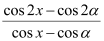# Thread: integrate........

1. ## integrate........2.Originally Posted by gaurav1292Full problem please? And what are we integrating in terms of? And is $\displaystyle \alpha$ a constant?

3. yeah a is constant..........integrate in terms of dx

4. I'd say split it up:

$\displaystyle \int\frac{cos2x - cos2\alpha}{cosx - cos\alpha}\,dx = \int\frac{cos2x}{cosx - cos\alpha}\,dx - \int\frac{cos2\alpha}{cosx - cos\alpha}\,dx$

Can you do it now?

5. i would say expand out cos2x - cos2a using the identity cos2x = cos^2 x - sin^2 x.

so on the top you would have cos^2 x - sin^2 x - cos^2 a + sin^2 a. group the cos terms and sin terms together and split up the fraction into:
(cos^2 x - cos^2 a) / (cos x - cos a) - (sin^2 x - sin^2 a) / (cos x - cos a)

factor cos^2 x - cos^2 a into (cosx + cosa)(cosx - cosa) so you can cancel out terms on the top and bottom so your first integral would become: integral of (cosx + cosa). now for the second integral, remember the identity sin^2 x + cos^2 x = 1. so add and subtract a cos^2 x term and add and subtract a cos^2 a term. on top you have: sin^2 x - sin^2 a + cos^2 x - cos^2 x + cos^2 a - cos^2 a. now group the terms like so: (sin^2 x + cos^2 x) - (sin^2 a + cos^2 a) - cos^2 x + cos^2 a. using the trig identity, you get 1 - 1 - cos^2 x + cos^2 a = -cos^2 x + cos^2 a. factor out a negative so that the negative sign bewtween the 2 integrals becomes a positive. you now have (cos^2 x - cos^2 a) / (cosx - cosa). same as before, factor out the top and cancel out common factors so you are left with the integral of (cosx + cosa).

so if you add that to the previous integral, you get 2(integral of (cosx + cosa)) = 2(sinx + xcosa) = 2sinx + 2x(cos a) + C

,

### integration of cos2x-cos2a/cos x-cos a

Click on a term to search for related topics.

#### Search Tags

integrate